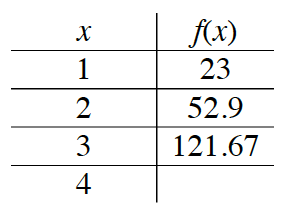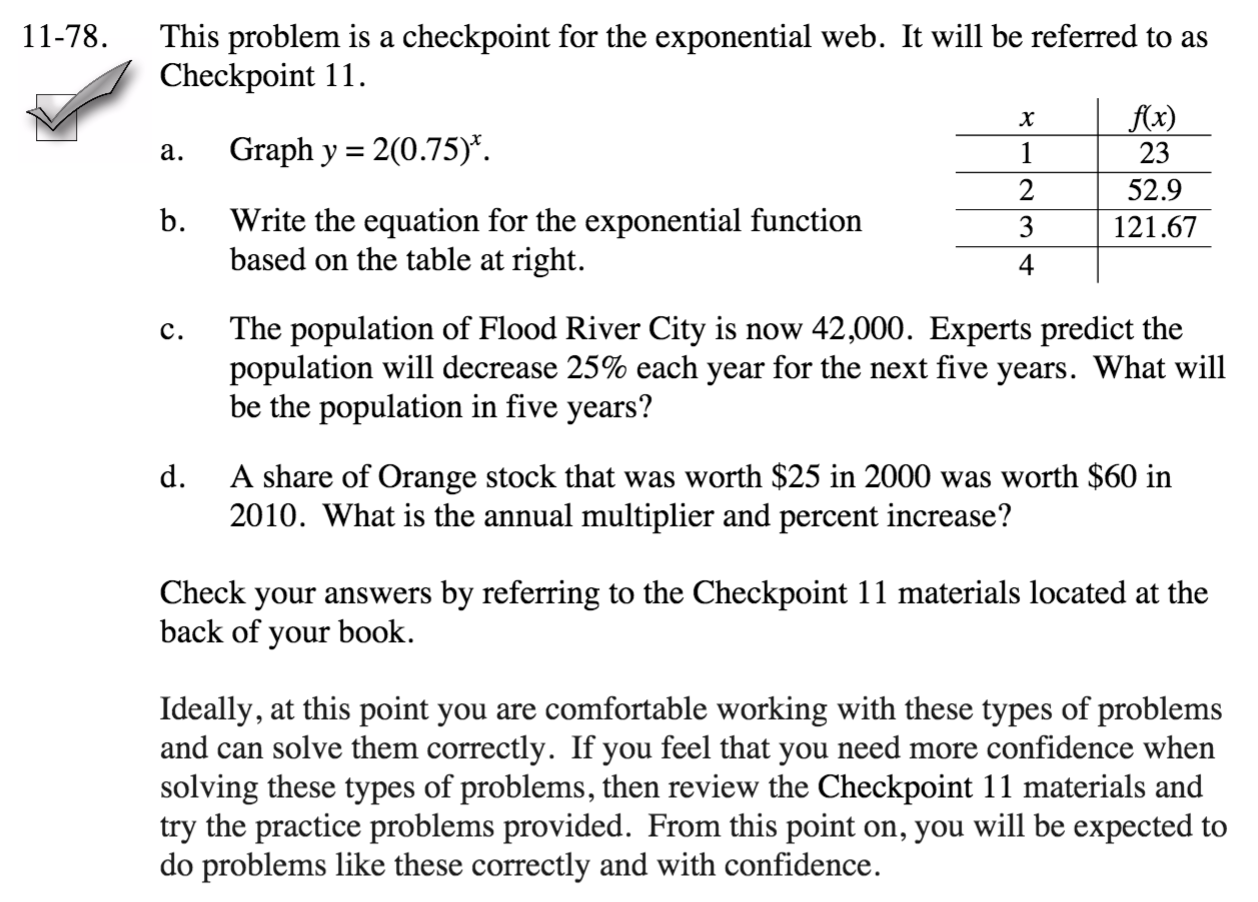Home > CC4 > Chapter 11 > Lesson 11.2.3 > Problem11-78

11-78.
1.This problem is a checkpoint for the exponential web. It will be referred to as Checkpoint 11. Homework Help ✎

1. Graph y = 2(0.75)x.

2. Write the equation for the exponential function based on the table below.3. The population of Flood River City is now 42,000. Experts predict the population will decrease 25% each year for the next five years. What will be the population in five years?

4. A share of Orange stock that was worth $25 in 2000 was worth$60 in 2010. What is the annual multiplier and percent increase?

2. Check your answers by referring to the Checkpoint 11 materials.

3. Ideally, at this point you are comfortable working with these types of problems and can solve them correctly. If you feel that you need more confidence when solving these types of problems, then review the Checkpoint 11 materials and try the practice problems provided. From this point on, you will be expected to do problems like these correctly and with confidence.Answers and extra practice are located in the back of your printed textbook or in the Reference Tab of your eBook.
If you have an eBook for INT1, login and then click the following link: Checkpoint 11 Answers and Practice